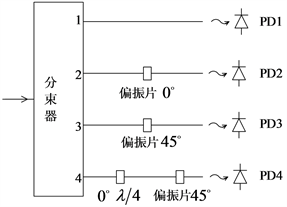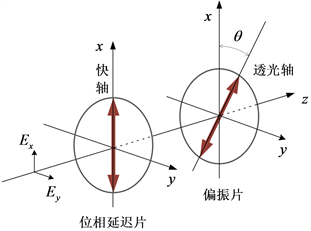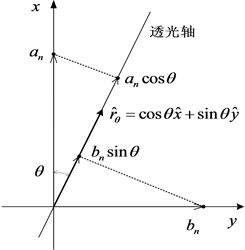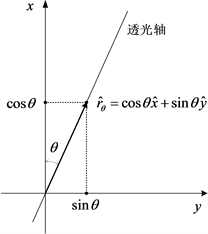# 对一种光子集合偏振态的理论研究A Theoretical Study of a Photon Set Polarization State

DOI: 10.12677/APP.2020.1012068, PDF, HTML, XML, 下载: 109  浏览: 636

Abstract: According to the quantum theory of light, the basic unit of light is photon. If the laser irradiates a uniform and smooth medium, all reflected photons have the same polarization state, and the result of the collective polarization state measurement is also the polarization state of a single photon. When measuring the polarization state of natural environment light, the result is the collective contribution of a large number of photons. In this case, the polarization state of each photon is different. In order to study the polarization state of light waves in the above-mentioned natural environment, this article mainly discusses a simple photon polarization state, where any photon polarization state will appear with a certain probability. In this article, the wave optics symbol is used in order to conform to the characterization of wave optics research polarization.

1. 引言

2. 理论计算

$A\left({u}_{1},{u}_{2},{u}_{3},{u}_{4},\cdots \right)$ (1)

${u}_{n}={a}_{n}\mathrm{exp}\left(j{\phi }_{nx}\right)\stackrel{^}{x}+{b}_{n}\mathrm{exp}\left(j{\phi }_{ny}\right)\stackrel{^}{y}$ (2)

${u}_{n}$ 为总的光子数量，其中 $\stackrel{^}{x}$$\stackrel{^}{y}$ 分别表示沿x轴和y轴的单位矢量，并且 ${a}_{n}^{2}+{b}_{n}^{2}=1$Figure 1. Principle of measuring polarization stateFigure 2. The orientation of the device (the third channel has a wave plate)Figure 3. Amplitude of light transmitted by polarizer

${{u}^{\prime }}_{n}=0.5{a}_{n}\mathrm{exp}\left(j{\phi }_{nx}\right)\stackrel{^}{x}+0.5{b}_{n}\mathrm{exp}\left(j{\phi }_{ny}\right)\stackrel{^}{y}$ (3)Figure 4. θ Unit vector decomposition of direction

${i}_{n1}=0.25\left({a}_{n}^{2}+{b}_{n}^{2}\right)$ (4)

${I}_{n1}={c}_{n}{i}_{n1}=0.25{c}_{n}\left({a}_{n}^{2}+{b}_{n}^{2}\right)$ (5)

${{\stackrel{\to }{u}}^{\prime }}_{n2}=0.5{a}_{n}\mathrm{exp}\left(j{\phi }_{nx}\right)\stackrel{^}{x}$ (6)

${i}_{n2}=0.25{a}_{n}^{2}$ (7)

${I}_{n2}=0.25{c}_{n}{a}_{n}^{2}$ (8)

${{u}^{″}}_{n3}=0.5\left[{a}_{n}\mathrm{cos}\theta \mathrm{exp}\left(j{\phi }_{nx}\right)+{b}_{n}\mathrm{sin}\theta \mathrm{exp}\left(j{\phi }_{ny}\right)\right]{\stackrel{^}{r}}_{\theta }$ (9)

${\stackrel{^}{r}}_{\theta }=\mathrm{cos}\theta \stackrel{^}{x}+\mathrm{sin}\theta \stackrel{^}{y}$ (10)

${{u}^{″}}_{n3}=0.25\sqrt{2}\left[{a}_{n}\mathrm{exp}\left(j{\phi }_{nx}\right)+{b}_{n}\mathrm{exp}\left(j{\phi }_{ny}\right)\right]{\stackrel{^}{r}}_{\theta }$ (11)

${i}_{n3}=0.125\left[{a}_{n}^{2}+{b}_{n}^{2}+2{a}_{n}{b}_{n}\mathrm{cos}{\delta }_{n}\right]$ (12)

${I}_{n3}={c}_{n}{i}_{n3}=0.125{c}_{n}\left[{a}_{n}^{2}+{b}_{n}^{2}+2{a}_{n}{b}_{n}\mathrm{cos}{\delta }_{n}\right]$ (13)

${{\stackrel{\to }{u}}^{\prime }}_{n4}=0.5{a}_{n}\mathrm{exp}\left(j{\phi }_{nx}\right)\stackrel{^}{x}+0.5{b}_{n}\mathrm{exp}\left[j\left({\phi }_{ny}+0.5\pi \right)\right]\stackrel{^}{y}$ (14)

${{u}^{″}}_{n4}=\left\{0.5{a}_{n}\mathrm{cos}\theta \mathrm{exp}\left(j{\phi }_{nx}\right)+0.5{b}_{n}\mathrm{sin}\theta \mathrm{exp}\left[j\left({\phi }_{ny}+0.5\pi \right)\right]\right\}{\stackrel{^}{r}}_{\theta }$ (15)

${{u}^{″}}_{n4}=0.25\sqrt{2}\left\{{a}_{n}\mathrm{exp}\left(j{\phi }_{nx}\right)+{b}_{n}\mathrm{exp}\left[j\left({\phi }_{ny}+0.5\pi \right)\right]\right\}{\stackrel{^}{r}}_{\theta }$ (16)

$\begin{array}{c}{i}_{n4}=0.125\left[{a}_{n}^{2}+{b}_{n}^{2}+2{a}_{n}{b}_{n}\mathrm{cos}\left({\phi }_{ny}+0.5\pi -{\phi }_{nx}\right)\right]\\ =0.125\left[{a}_{n}^{2}+{b}_{n}^{2}-2{a}_{n}{b}_{n}\mathrm{sin}\left({\phi }_{ny}-{\phi }_{nx}\right)\right]\\ =0.125\left[{a}_{n}^{2}+{b}_{n}^{2}-2{a}_{n}{b}_{n}\mathrm{sin}{\delta }_{n}\right]\end{array}$ (17)

${I}_{n4}=0.125{c}_{n}\left[{a}_{n}^{2}+{b}_{n}^{2}-2{a}_{n}{b}_{n}\mathrm{sin}{\delta }_{n}\right]$ (18)

${s}_{n0}={I}_{n1}=0.25{c}_{n}\left({a}_{n}^{2}+{b}_{n}^{2}\right)$ (19)

${s}_{n1}=2{I}_{n2}-{I}_{n1}=0.5{c}_{n}{a}_{n}^{2}-0.25{c}_{n}\left({a}_{n}^{2}+{b}_{n}^{2}\right)=0.25{c}_{n}\left({a}_{n}^{2}-{b}_{n}^{2}\right)$ (20)

${s}_{n2}=2{I}_{n3}-{I}_{n1}=0.25{c}_{n}\left({a}_{n}^{2}+{b}_{n}^{2}+2{a}_{n}{b}_{n}\mathrm{cos}{\delta }_{n}\right)-0.25{c}_{n}\left({a}_{n}^{2}+{b}_{n}^{2}\right)=0.5{c}_{n}{a}_{n}{b}_{n}\mathrm{cos}{\delta }_{n}$ (21)

${s}_{n3}={I}_{n1}-2{I}_{n4}=0.25{c}_{n}\left({a}_{n}^{2}+{b}_{n}^{2}\right)-0.25{c}_{n}\left({a}_{n}^{2}+{b}_{n}^{2}-2{a}_{n}{b}_{n}\mathrm{sin}{\delta }_{n}\right)=0.5{c}_{n}{a}_{n}{b}_{n}\mathrm{sin}{\delta }_{n}$ (22)

3. 光子集合与Stokes关系

${I}_{1}={\sum }_{n}{I}_{n1}=0.25{\sum }_{n}{c}_{n}\left({a}_{n}^{2}+{b}_{n}^{2}\right)$ (23)

${I}_{2}={\sum }_{n}{I}_{n}{}_{2}=0.25{\sum }_{n}{c}_{n}{a}_{n}^{2}$ (24)

${I}_{3}={\sum }_{n}{I}_{n3}=0.125{\sum }_{n}{c}_{n}\left[{a}_{n}^{2}+{b}_{n}^{2}+2{a}_{n}{b}_{n}\mathrm{cos}{\delta }_{n}\right]$ (25)

${I}_{4}={\sum }_{n}{I}_{n4}=0.125{\sum }_{n}{c}_{n}\left[{a}_{n}^{2}+{b}_{n}^{2}-2{a}_{n}{b}_{n}\mathrm{sin}{\delta }_{n}\right]$ (26)

(22)式至(25)式探测器输出信号 ${I}_{1}$${I}_{2}$${I}_{3}$${I}_{4}$ 为光子集合的贡献。此时系统给出的斯托克斯参量为

${S}_{0}={\sum }_{n}{I}_{n1}=0.25{\sum }_{n}{c}_{n}\left({a}_{n}^{2}+{b}_{n}^{2}\right)={\sum }_{n}{s}_{n0}$ (27)

${S}_{1}={\sum }_{n}\left(2{I}_{n2}-{I}_{n1}\right)=0.25{\sum }_{n}{c}_{n}\left({a}_{n}^{2}-{b}_{n}^{2}\right)={\sum }_{n}{s}_{n1}$ (28)

${S}_{2}={\sum }_{n}\left(2{I}_{n3}-{I}_{n1}\right)=0.5{\sum }_{n}{c}_{n}{a}_{n}{b}_{n}\mathrm{cos}{\delta }_{n}={\sum }_{n}{s}_{n2}$ (29)

${S}_{3}={\sum }_{n}\left({I}_{n1}-2{I}_{n4}\right)=0.5{\sum }_{n}{c}_{n}{a}_{n}{b}_{n}\mathrm{sin}{\delta }_{n}={\sum }_{n}{s}_{n3}$ (30)

4. 结论

${{S}^{\prime }}_{0}={\sum }_{n}{s}_{n0}=0.25{c}_{1}\left({a}_{1}^{2}+{b}_{1}^{2}\right)+0.25{c}_{1}\left({a}_{2}^{2}+{b}_{2}^{2}\right)$ (31)

${{S}^{\prime }}_{1}={\sum }_{n}{s}_{n1}=0.25{c}_{1}\left({a}_{1}^{2}-{b}_{1}^{2}\right)+0.25{c}_{1}\left({a}_{2}^{2}-{b}_{2}^{2}\right)$ (32)

${{S}^{\prime }}_{2}={\sum }_{n}{s}_{n2}=0.5{c}_{1}{a}_{1}{b}_{1}\mathrm{cos}{\delta }_{1}+0.5{c}_{2}{a}_{2}{b}_{2}\mathrm{cos}{\delta }_{2}$ (33)

${{S}^{\prime }}_{3}={\sum }_{n}{s}_{n3}=0.5{c}_{1}{a}_{1}{b}_{1}\mathrm{sin}{\delta }_{1}+0.5{c}_{2}{a}_{2}{b}_{2}\mathrm{sin}{\delta }_{2}$ (34)

${{S}^{″}}_{0}={\sum }_{n}{s}_{n0}=0.25{c}_{3}\left({a}_{3}^{2}+{b}_{3}^{2}\right)+0.25{c}_{4}\left({a}_{4}^{2}+{b}_{4}^{2}\right)$ (35)

${{S}^{″}}_{1}={\sum }_{n}{s}_{n1}=0.25{c}_{3}\left({a}_{3}^{2}-{b}_{3}^{2}\right)+0.25{c}_{4}\left({a}_{4}^{2}-{b}_{4}^{2}\right)$ (36)

${{S}^{″}}_{2}={\sum }_{n}{s}_{n2}=0.5{c}_{3}{a}_{3}{b}_{3}\mathrm{cos}{\delta }_{3}+0.5{c}_{4}{a}_{4}{b}_{4}\mathrm{cos}{\delta }_{4}$ (37)

${{S}^{″}}_{3}={\sum }_{n}{s}_{n3}=0.5{c}_{3}{a}_{3}{b}_{3}\mathrm{sin}{\delta }_{3}+0.5{c}_{4}{a}_{4}{b}_{4}\mathrm{sin}{\delta }_{4}$ (38)

NOTES

*通讯作者。

  施志华. 成像偏振测量技术及其应用[J]. 红外, 2002(4): 1-5.  Namer, E. and Scheclmer, Y.Y. (2005) Advanced Visibility Improvement Based on Polarization Filtered Images. Proceeding of SPIE, 5888, Article ID: 588805. https://doi.org/10.1117/12.617464  Stankiewicz, K.A. (2005) Crop Discrimination on Envisat Asar Images Acquired Inalternating Polarization Mode. Proceeding of SPIE, 5976, Article ID: 597615. https://doi.org/10.1117/12.634715  Cheng, J.Y. and Chen, Q.S. (2006) An Ultrafast Phase Modulator for 3D Imaging. Proceeding of SPIE, 6068, Article ID: 60680L. https://doi.org/10.1117/12.643160  Egan, W.G. and Duggin, M.J. (2002) Synthesis of Optical Polarization Signatures of Military Aircraft. Proceeding of SPIE, 4481. https://doi.org/10.1117/12.452888  Taylor, J.S., Davis, P.S. and Wolff, L.B. (2002) Underwater Partial Polari-zation Signatures from the Shallow Water Real-Time Imaging Polarimeter (SHRIMP). OCEANS'02 MTS/IEEE, Biloxi, MI, 29-31 October 2002, 1526-1534. https://doi.org/10.1109/OCEANS.2002.1191863  吕鑫, 蒋卓勤, 李迎春. 偏振态的高速实时测量方法的研究[J]. 光学技术, 2010, 6(6): 866-870.  马号, 熊剑, 郭杭,等. 基于大气偏振光特性辅助定向的自主导航方法[J]. 应用光学, 2016, 37(2): 162-167.  于洵, 杨烨, 姜旭, 等. 基于偏振光谱成像的目标识别方法研究[J]. 应用光学, 2016, 37(4): 537-541.  王玉波, 杨江涛, 唐军, 等. 基于大气偏振光的航向角度探测系统[J]. 兵器装备工程学报, 2019, 40(3): 127-131.  罗英达, 陈哲. 偏振光斯托克斯参量测量原理与测量方法设计[J]. 激光与光电子进展, 2009(6): 54-58.